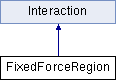ParM  parm A molecular dynamics library
FixedForceRegion Class Reference

#include <interaction.hpp>

Inheritance diagram for FixedForceRegion:## Public Member Functions

FixedForceRegion (vector< FixedForceRegionAtom > atoms=vector< FixedForceRegionAtom >())

void add (FixedForceRegionAtom a)

void add (AtomID a, Vec dir, vector< flt > bound, vector< flt > F)

uint size () const

flt energy (Box &box)
Potential energy due to this Interaction. More...

void set_forces (Box &box)

flt pressure (Box &box)
Partial pressure due to this Interaction. More...Public Member Functions inherited from Interaction
virtual flt set_forces_get_pressure (Box &box)
Set forces (Atom.f) and return $$P = \sum_{\left<i,j \right>} \vec r_{ij} \cdot \vec F_{ij}$$ at the same time (see pressure()). More...

virtual Matrix stress (Box &box)
The force-moment tensor for the current simulation: More...

virtual ~Interaction ()

## Protected Attributes

vector< FixedForceRegionAtomatoms

## Constructor & Destructor Documentation

 FixedForceRegion::FixedForceRegion ( vector< FixedForceRegionAtom > atoms = vector() )
inline

## Member Function Documentation

 void FixedForceRegion::add ( FixedForceRegionAtom a )
inline
 void FixedForceRegion::add ( AtomID a, Vec dir, vector< flt > bound, vector< flt > F )
inline
 flt FixedForceRegion::energy ( Box & box )
inlinevirtual

Potential energy due to this Interaction.

Implements Interaction.

 flt FixedForceRegion::pressure ( Box & box )
inlinevirtual

Partial pressure due to this Interaction.

$$P = \sum_{\left<i,j \right>} \vec r_{ij} \cdot \vec F_{ij}$$, or equivalently $$P = \sum_i \vec r_i \cdot \vec F_i$$

Note that the full pressure involves all interactions and temperature, and needs to be normalized by $$\frac{1}{dV}$$ where $$d$$ is the number of dimensions and $$V$$ is the volume.

Implements Interaction.

 void FixedForceRegion::set_forces ( Box & box )
inlinevirtual

Implements Interaction.

 uint FixedForceRegion::size ( ) const
inline

## Member Data Documentation

 vector FixedForceRegion::atoms
protected

The documentation for this class was generated from the following file: